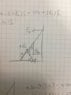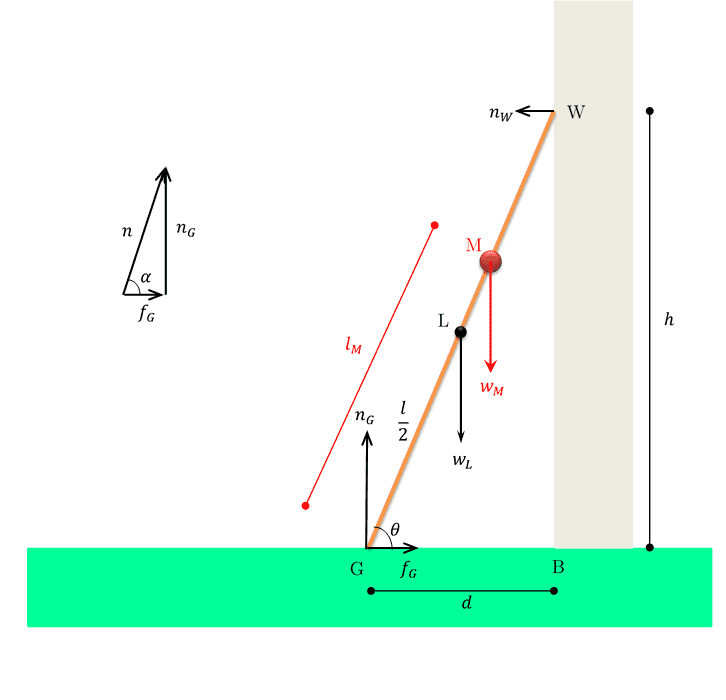# A ladder against a wall problem

• JessicaHelena

## Homework Statement

A 70 kg window cleaner uses a 16 kg ladder that is 5.6 m long. He places one end on the ground 2.0 m from a wall, rests the upper end against a cracked window, and climbs the ladder. He is 3.5 m up along the ladder when the window breaks. Neglect friction between the ladder and window and assume that the base of the ladder does not slip.
(a) When the window is on the verge of breaking, what is the magnitude of the force on the window from the ladder?
(b) When the window is on the verge of breaking, what is the magnitude of the force on the ladder from the ground?
(c) When the window is on the verge of breaking, what is the angle of that force on the ladder?

tau_net = 0.
tau = r x F

## The Attempt at a Solution

I was able to get part (a), which was 193.914N. But I don't get part (b). I drew a forces diagram as shown (attached file). I resolved the force on the ladder from the ground into horizontal and vertical components because otherwise this force will have no torque, and how could I calculate the force then?
[FONT=verdana, helvetica, sans-serif]I count ccw as positive and cw as negative, and the system is not moving so net torque is 0.

So I put the origin where the ladder meets the vertical wall. Then there will only be 1 F_N, and furthermore, only one ccw torque: F_N(x). Since the angle it makes is the complement of theta, I can use cos (theta) instead of sin theta.

The full equation would then look something like this:
tau_net = F_N(x)*5.6*cos(theta) - F_N(y)*5.6*sin(theta) - F_gl*5.6/2*sin(theta) - F_gp*(5.6-3.5)*sin(theta) = 0.
We can calculate theta since we're given the hypotenuse 5.6 and one of the legs 2.

But this gives me two unknowns — F_N(x) and F_N(y). How can I solve this?
Also, for (c), I've no idea which force they're talking about...

Any help would really be appreciated!
[/FONT]

#### Attachments

•IMG_0309.JPG
26.6 KB · Views: 459

tau_net = 0.

## The Attempt at a Solution

tau_net = F_N(x)*5.6*cos(theta) - F_N(y)*5.6*sin(theta) - F_gl*5.6/2*sin(theta) - F_gp*(5.6-3.5)*sin(theta) = 0.

Why do you want to calculate the force from the ground on the ladder with the equlibrium of torques? Wouldn't it be easier to calculate it from the equilibrium of forces (since you've calculated the force from the window on the ladder in a))?

I was able to get part (a), which was 193.914N.
Exactly how did you do that? You must have calculated the torques about the point where the ladder makes contact with the ground. For parts (b) & (c) you need to balance forces in the horizontal and vertical directions, 2 equations 2 unknowns. For part (c) they are talking about the resultant force that the ground exerts on the ladder. Contrary to popular belief, it is not necessarily along the ladder, which is what you show in your diagram.

QUICK ANALYSIS

NOTATION

; is a comment separator, used when helpful to clarity
units not shown
significant figures inconsistent; quickly done to illustrate method

ASSERTIONS AND ASSUMPTIONS AND REMINDERS
Window only exerts normal force on ladder.
Gravitational forces on person act through one point 3.5 m up the ladder.
Point O is the base of ladder.

GEOMETRY
LADDER_TOPY = SQRT(5.6^2 - 2^2) = 5.23
COS(2/5.6) = COS(69.1) =.36
PERSON_POSITION is 3.5; more than halfway
PERSON_X = 3.5*.36 = 1.26
PERSON_Y = SQRT (3.5^2-1.26^2) = 3.27
ix> unit vector right (three character notation)
iy> unit vector up
iz> = ix> X iy> unit vector out of paper; X indicates cross product

FORCES AS VECTORS ON (LADDER + PERSON)

F_G_PERSON = -70(9.8) iy> at point 1.26 ix> + 3.27 iy> ; gravity
F_G_LADDER = -16(9.8) iy> at point 1.0 ix> + 2.61 iy> ; halfway up ladder ;gravity
F_WINDOW = F_WX ix> ; force of window on ladder
F_BASE = F_BX ix> + F_BY iy> AT O; force of ground on ladder

EQUILIBRIUM (SCALAR EQUATIONS)

F_G_PERSON, F_G_LADDER, F_WINDOW, F_BASE, 4 force vectors: 3 unknowns
SUMFX: F_WX + F_BX = 0
SUMFY: F_BY -70(9.8)-16(9.8) ==> F_BY = 86(9.8) = 842.8
Remember Point O is the base of ladder; take moments about O
MOMZO = -70(1.26)9.8 -16(1.0)(9.8) - F_WX(5.23) = 0
MOMZO = -104.2*9.8 -F_WX(5.23) = 0
After solving
F_WX = -195.25
F_BX = 195.25

FORCES AS VECTORS
F_G_PERSON = -686 iy>
F_WINDOW = -195.25 ix>
F_BASE = 195.25 ix> + 843 iy> ==> MAG = 865

PARTIAL CHECK
{0, -686} + {0, -157} + {-195.25, 0} + {195.25, 843} = {0, 0}

Last edited:
F_BASE = 195.25 ix> + 843 iy> ==> MAG = 865
The above is the answer to part (b).
Can you do part (c)? I assume you are asked to find the angle between F_BASE and the ladder.The diagram shows the the forces and distances.

(a) Using ##\Sigma \vec F = 0## for the ladder, we have the two equations ##\underline{w_L+w_M=n_G}## and ##\underline{f_G = n_W}##.

Taking moments about the ground ##G##, we have ##w_L d/2+W_M l_M \cos \theta = n_W h## which leads to ##\mathbf{n_W} = \frac{w_L d/2+w_M l_M \cos\theta}{h} = \frac{16 \times 9.8 \times 1+70\times 9.8\times 3.5\times \cos 69.1^{\circ}}{5.6 \sin 69.1^{\circ}} = \mathbf{193.69 \text{N}}## .

From the underlined equations above, we get ##\mathbf{f_G = 193.69 \text{N}}## and ##\mathbf{n_G} = (16+70)\times 9.8 = \mathbf{842.8 \text{N}}##.

(b) From the diagram above (see inlet), we find that the force of the ground on the ladder ##\mathbf{n} = \sqrt{n_G^2+f_G^2} = \sqrt{842.8^2+193.69^2} = \mathbf{864.77 \text{N}}##.

(c) The angle that force from the ground makes with the horizontal : ##\boldsymbol{\alpha} = \tan^{-1} \frac{n_G}{f_G} = \mathbf{77.06^{\circ}}##.

Reflection :

1. Argue why ##\alpha > \theta##. One way to do that is to show that if the two angles were the same then the ladder will not be in equilibrium.

2. Let the friction due to the window (wall) be some ##f_W##. Can you solve for the (four) forces?